Publicité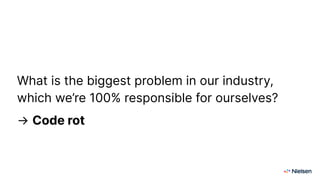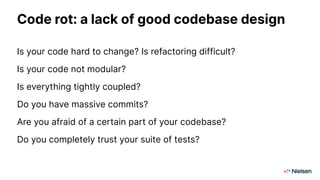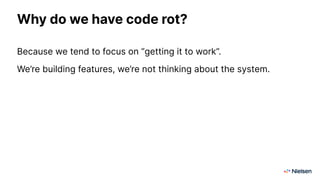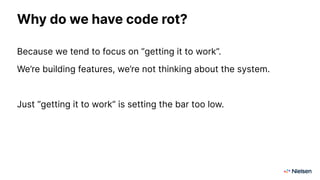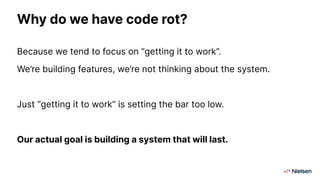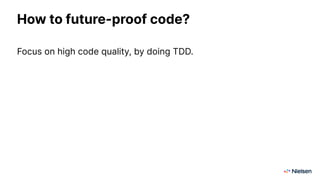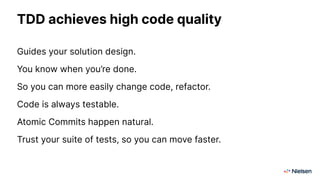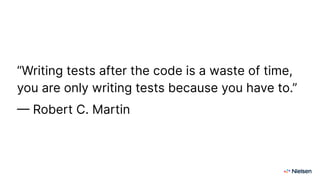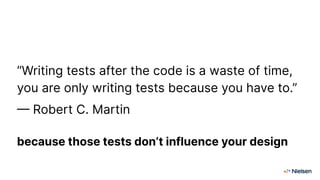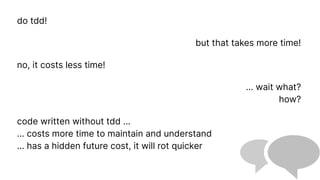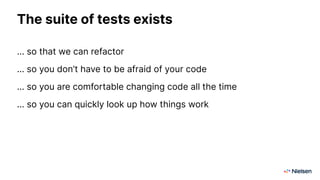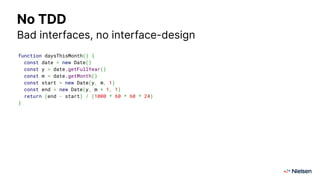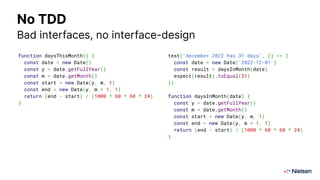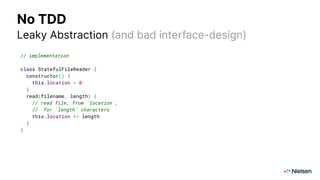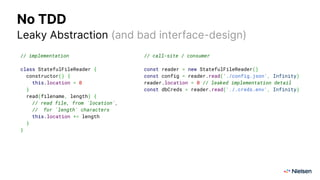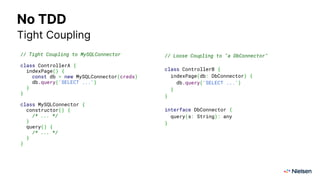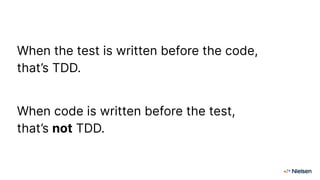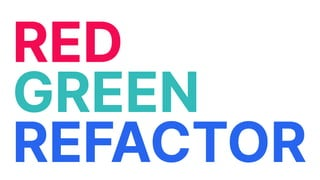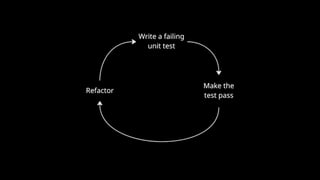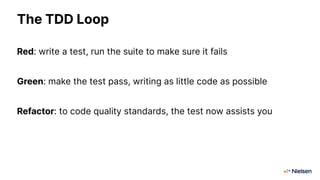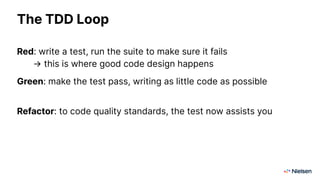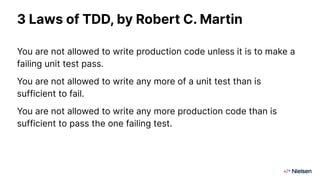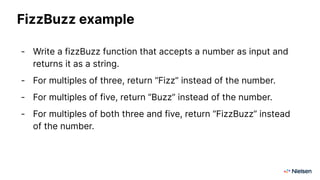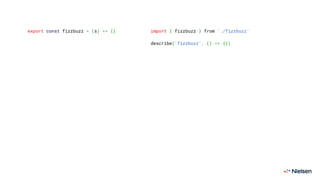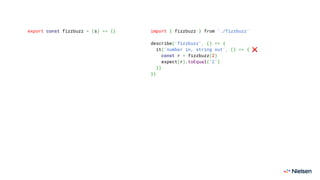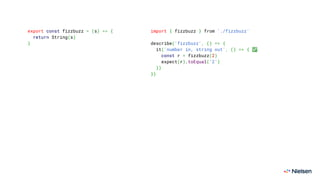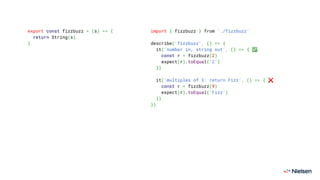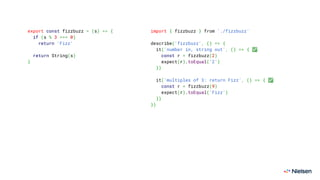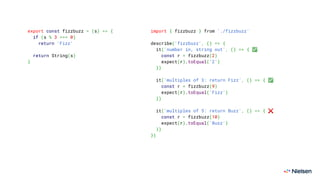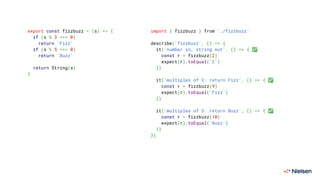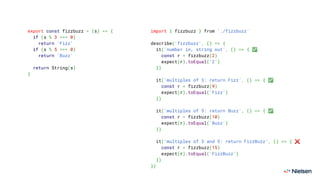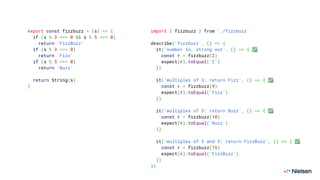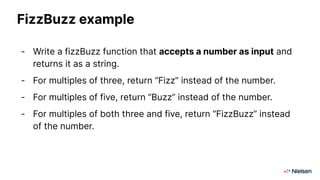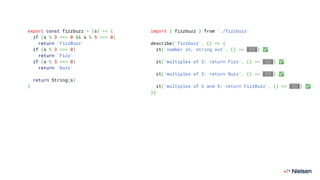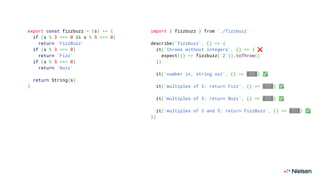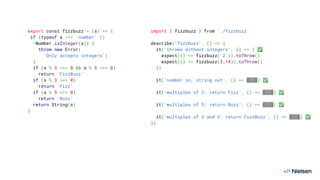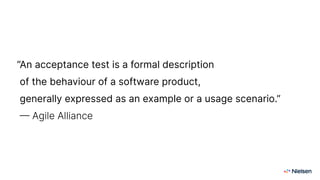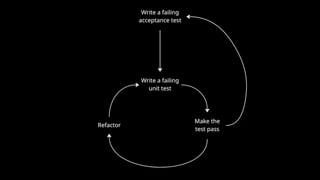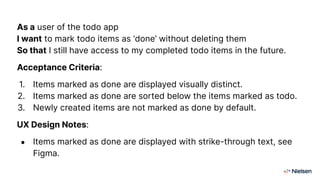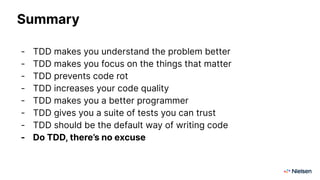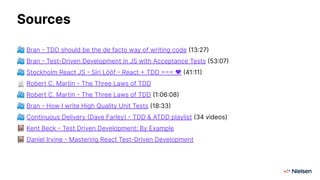1 sur 54
Publicité

### Test-driven Development (TDD)

1. TDD
2. Hi! I’m Bran this is my face → https://bran.name/ youtube.com/@branvandermeer twitter.com/branvandermeer
3. WHY?
4. What is the biggest problem in our industry, which we’re 100% responsible for ourselves?
5. What is the biggest problem in our industry, which we’re 100% responsible for ourselves? → Code rot
6. What is the biggest problem in our industry, which we’re 100% responsible for ourselves? → Code rot (a.k.a. low code quality)
7. Code rot: a lack of good codebase design Is your code hard to change? Is refactoring difficult? Is your code not modular? Is everything tightly coupled? Do you have massive commits? Are you afraid of a certain part of your codebase? Do you completely trust your suite of tests?
8. Why do we have code rot? Because we tend to focus on “getting it to work”. We’re building features, we’re not thinking about the system.
9. Why do we have code rot? Because we tend to focus on “getting it to work”. We’re building features, we’re not thinking about the system. Just “getting it to work” is setting the bar too low.
10. Why do we have code rot? Because we tend to focus on “getting it to work”. We’re building features, we’re not thinking about the system. Just “getting it to work” is setting the bar too low. Our actual goal is building a system that will last.
11. How to future-proof code? Focus on high code quality, by doing TDD.
12. What is high code quality? Great interface-design (the concept of an interface) No leaky abstractions Great separation of concerns Reasonable coupling (more loose coupling than tight coupling) High testability Little mocks, the right amount
13. TDD achieves high code quality Guides your solution design. You know when you’re done. So you can more easily change code, refactor. Code is always testable. Atomic Commits happen natural. Trust your suite of tests, so you can move faster.
14. “Writing tests after the code is a waste of time, you are only writing tests because you have to.” — Robert C. Martin
15. “Writing tests after the code is a waste of time, you are only writing tests because you have to.” — Robert C. Martin because those tests don’t influence your design
16. do tdd! why? it increases code quality … huh? … how? it forces you to ‘design’ your code but I design my code! but you dont have a workflow that enforces good design
17. do tdd! but that takes more time! no, it costs less time! ... wait what? how? code written without tdd … … costs more time to maintain and understand … has a hidden future cost, it will rot quicker
18. do tdd! but i dont know how! then learn its just a skill you have to learn new things all the time anyway ok, fair enough
19. The suite of tests exists … so that we can refactor … so you don’t have to be afraid of your code … so you are comfortable changing code all the time … so you can quickly look up how things work
20. function daysThisMonth() { const date = new Date() const y = date.getFullYear() const m = date.getMonth() const start = new Date(y, m, 1) const end = new Date(y, m + 1, 1) return (end - start) / (1000 * 60 * 60 * 24) } No TDD Bad interfaces, no interface-design
21. function daysThisMonth() { const date = new Date() const y = date.getFullYear() const m = date.getMonth() const start = new Date(y, m, 1) const end = new Date(y, m + 1, 1) return (end - start) / (1000 * 60 * 60 * 24) } test('december 2022 has 31 days', () => { const date = new Date('2022-12-01') const result = daysInMonth(date) expect(result).toEqual(31) }) function daysInMonth(date) { const y = date.getFullYear() const m = date.getMonth() const start = new Date(y, m, 1) const end = new Date(y, m + 1, 1) return (end - start) / (1000 * 60 * 60 * 24) } No TDD Bad interfaces, no interface-design
22. No TDD Leaky Abstraction (and bad interface-design) // implementation class StatefulFileReader { constructor() { this.location = 0 } read(filename, length) { // read file, from `location`, // for `length` characters this.location += length } }
24. No TDD Tight Coupling // Tight Coupling to MySQLConnector class ControllerA { indexPage() { const db = new MySQLConnector(creds) db.query('SELECT ...') } } class MySQLConnector { constructor() { /* ... */ } query() { /* ... */ } }
25. // Loose Coupling to "a DbConnector" class ControllerB { indexPage(db: DbConnector) { db.query('SELECT ...') } } interface DbConnector { query(s: String): any } No TDD Tight Coupling // Tight Coupling to MySQLConnector class ControllerA { indexPage() { const db = new MySQLConnector(creds) db.query('SELECT ...') } } class MySQLConnector { constructor() { /* ... */ } query() { /* ... */ } }
26. HOW?
27. When the test is written before the code, that’s TDD. When code is written before the test, that’s not TDD.
28. RED GREEN REFACTOR
29. The TDD Loop Red: write a test, run the suite to make sure it fails Green: make the test pass, writing as little code as possible Refactor: to code quality standards, the test now assists you
30. The TDD Loop Red: write a test, run the suite to make sure it fails → this is where good code design happens Green: make the test pass, writing as little code as possible Refactor: to code quality standards, the test now assists you
31. 3 Laws of TDD, by Robert C. Martin You are not allowed to write production code unless it is to make a failing unit test pass. You are not allowed to write any more of a unit test than is sufficient to fail. You are not allowed to write any more production code than is sufficient to pass the one failing test.
32. Requirements … will enable you to write good tests … will prevent you from starting when they’re vague (because you won’t know what test to write) … as acceptance criteria can become your test cases
33. FizzBuzz example - Write a fizzBuzz function that accepts a number as input and returns it as a string. - For multiples of three, return “Fizz” instead of the number. - For multiples of five, return “Buzz” instead of the number. - For multiples of both three and five, return “FizzBuzz” instead of the number.
34. export const fizzbuzz = (s) => {} import { fizzbuzz } from './fizzbuzz' describe('fizzbuzz', () => {})
35. export const fizzbuzz = (s) => {} import { fizzbuzz } from './fizzbuzz' describe('fizzbuzz', () => { it('number in, string out', () => { ❌ const r = fizzbuzz(2) expect(r).toEqual('2') }) })
36. export const fizzbuzz = (s) => { return String(s) } import { fizzbuzz } from './fizzbuzz' describe('fizzbuzz', () => { it('number in, string out', () => { ✅ const r = fizzbuzz(2) expect(r).toEqual('2') }) })
37. export const fizzbuzz = (s) => { return String(s) } import { fizzbuzz } from './fizzbuzz' describe('fizzbuzz', () => { it('number in, string out', () => { ✅ const r = fizzbuzz(2) expect(r).toEqual('2') }) it('multiples of 3: return Fizz', () => { ❌ const r = fizzbuzz(9) expect(r).toEqual('Fizz') }) })
38. export const fizzbuzz = (s) => { if (s % 3 === 0) return 'Fizz' return String(s) } import { fizzbuzz } from './fizzbuzz' describe('fizzbuzz', () => { it('number in, string out', () => { ✅ const r = fizzbuzz(2) expect(r).toEqual('2') }) it('multiples of 3: return Fizz', () => { ✅ const r = fizzbuzz(9) expect(r).toEqual('Fizz') }) })
39. export const fizzbuzz = (s) => { if (s % 3 === 0) return 'Fizz' return String(s) } import { fizzbuzz } from './fizzbuzz' describe('fizzbuzz', () => { it('number in, string out', () => { ✅ const r = fizzbuzz(2) expect(r).toEqual('2') }) it('multiples of 3: return Fizz', () => { ✅ const r = fizzbuzz(9) expect(r).toEqual('Fizz') }) it('multiples of 5: return Buzz', () => { ❌ const r = fizzbuzz(10) expect(r).toEqual('Buzz') }) })
40. export const fizzbuzz = (s) => { if (s % 3 === 0) return 'Fizz' if (s % 5 === 0) return 'Buzz' return String(s) } import { fizzbuzz } from './fizzbuzz' describe('fizzbuzz', () => { it('number in, string out', () => { ✅ const r = fizzbuzz(2) expect(r).toEqual('2') }) it('multiples of 3: return Fizz', () => { ✅ const r = fizzbuzz(9) expect(r).toEqual('Fizz') }) it('multiples of 5: return Buzz', () => { ✅ const r = fizzbuzz(10) expect(r).toEqual('Buzz') }) })
41. export const fizzbuzz = (s) => { if (s % 3 === 0) return 'Fizz' if (s % 5 === 0) return 'Buzz' return String(s) } import { fizzbuzz } from './fizzbuzz' describe('fizzbuzz', () => { it('number in, string out', () => { ✅ const r = fizzbuzz(2) expect(r).toEqual('2') }) it('multiples of 3: return Fizz', () => { ✅ const r = fizzbuzz(9) expect(r).toEqual('Fizz') }) it('multiples of 5: return Buzz', () => { ✅ const r = fizzbuzz(10) expect(r).toEqual('Buzz') }) it('multiples of 3 and 5: return FizzBuzz', () => { ❌ const r = fizzbuzz(15) expect(r).toEqual('FizzBuzz') }) })
42. export const fizzbuzz = (s) => { if (s % 3 === 0 && s % 5 === 0) return 'FizzBuzz' if (s % 3 === 0) return 'Fizz' if (s % 5 === 0) return 'Buzz' return String(s) } import { fizzbuzz } from './fizzbuzz' describe('fizzbuzz', () => { it('number in, string out', () => { ✅ const r = fizzbuzz(2) expect(r).toEqual('2') }) it('multiples of 3: return Fizz', () => { ✅ const r = fizzbuzz(9) expect(r).toEqual('Fizz') }) it('multiples of 5: return Buzz', () => { ✅ const r = fizzbuzz(10) expect(r).toEqual('Buzz') }) it('multiples of 3 and 5: return FizzBuzz', () => { ✅ const r = fizzbuzz(15) expect(r).toEqual('FizzBuzz') }) })
43. FizzBuzz example - Write a fizzBuzz function that accepts a number as input and returns it as a string. - For multiples of three, return “Fizz” instead of the number. - For multiples of five, return “Buzz” instead of the number. - For multiples of both three and five, return “FizzBuzz” instead of the number.
44. FizzBuzz example - Write a fizzBuzz function that accepts a number as input and returns it as a string. - For multiples of three, return “Fizz” instead of the number. - For multiples of five, return “Buzz” instead of the number. - For multiples of both three and five, return “FizzBuzz” instead of the number.
45. export const fizzbuzz = (s) => { if (s % 3 === 0 && s % 5 === 0) return 'FizzBuzz' if (s % 3 === 0) return 'Fizz' if (s % 5 === 0) return 'Buzz' return String(s) } import { fizzbuzz } from './fizzbuzz' describe('fizzbuzz', () => { it('number in, string out', () => {..}) ✅ it('multiples of 3: return Fizz', () => {..}) ✅ it('multiples of 5: return Buzz', () => {..}) ✅ it('multiples of 3 and 5: return FizzBuzz', () => {..}) ✅ })
46. export const fizzbuzz = (s) => { if (s % 3 === 0 && s % 5 === 0) return 'FizzBuzz' if (s % 3 === 0) return 'Fizz' if (s % 5 === 0) return 'Buzz' return String(s) } import { fizzbuzz } from './fizzbuzz' describe('fizzbuzz', () => { it('throws without integers', () => { ❌ expect(() => fizzbuzz('2')).toThrow() }) it('number in, string out', () => {..}) ✅ it('multiples of 3: return Fizz', () => {..}) ✅ it('multiples of 5: return Buzz', () => {..}) ✅ it('multiples of 3 and 5: return FizzBuzz', () => {..}) ✅ })
47. export const fizzbuzz = (s) => { if (typeof s !== 'number' || !Number.isInteger(s)) { throw new Error( 'Only accepts integers') } if (s % 3 === 0 && s % 5 === 0) return 'FizzBuzz' if (s % 3 === 0) return 'Fizz' if (s % 5 === 0) return 'Buzz' return String(s) } import { fizzbuzz } from './fizzbuzz' describe('fizzbuzz', () => { it('throws without integers', () => { ✅ expect(() => fizzbuzz('2')).toThrow() expect(() => fizzbuzz(3.14)).toThrow() }) it('number in, string out', () => {..}) ✅ it('multiples of 3: return Fizz', () => {..}) ✅ it('multiples of 5: return Buzz', () => {..}) ✅ it('multiples of 3 and 5: return FizzBuzz', () => {..}) ✅ })
48. ATDD
49. “An acceptance test is a formal description of the behaviour of a software product, generally expressed as an example or a usage scenario.” — Agile Alliance
50. As a user of the todo app I want to mark todo items as ‘done’ without deleting them So that I still have access to my completed todo items in the future. Acceptance Criteria: 1. Items marked as done are displayed visually distinct. 2. Items marked as done are sorted below the items marked as todo. 3. Newly created items are not marked as done by default. UX Design Notes: ● Items marked as done are displayed with strike-through text, see Figma.
51. Summary - TDD makes you understand the problem better - TDD makes you focus on the things that matter - TDD prevents code rot - TDD increases your code quality - TDD makes you a better programmer - TDD gives you a suite of tests you can trust - TDD should be the default way of writing code - Do TDD, there’s no excuse
52. Sources 📽 Bran - TDD should be the de facto way of writing code (13:27) 📽 Bran - Test-Driven Development in JS with Acceptance Tests (53:07) 📽 Stockholm React JS - Siri Lööf - React + TDD === ♥ (41:11) 📄 Robert C. Martin - The Three Laws of TDD 📽 Robert C. Martin - The Three Laws of TDD (1:06:08) 📽 Bran - How I write High Quality Unit Tests (18:33) 📽 Continuous Delivery (Dave Farley) - TDD & ATDD playlist (34 videos) 📕 Kent Beck - Test Driven Development: By Example 📕 Daniel Irvine - Mastering React Test-Driven Development
Publicité